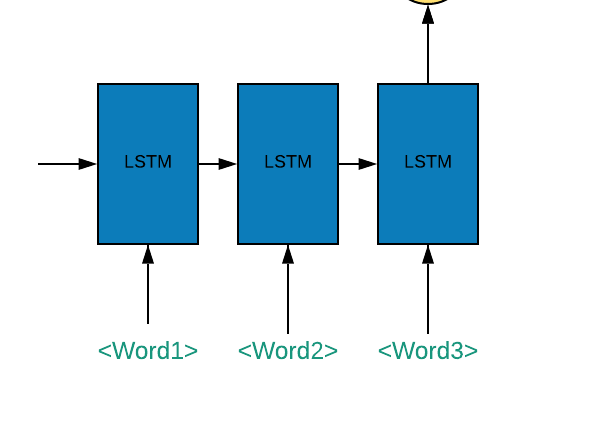# How to create many to one LSTM of this form?

I am trying to create a 3 to 1 LSTMThe LSTM must take sequence of 3 words, each embedded vector of size 100
So, my input size is (batch_size, 3, 100). So, what is the way to achieve this? I am new to pytorch, so, please help me with this

Usually LSTMs are used for inputs with varying length, for exactly 3 inputs with a total vector size of 300 you can just use a few nn.Linear layers.

Nevertheless, if you want to use an LSTM, you want the following:

Your final code will look like this:

``````model = torch.nn.LSTM(input_size=100, hidden_size=128, num_layers=1)
output = model(input)[-1]
``````

isn’t output the first element of the tuple returned as per doc? the o/p has shape (seq_len, batch, num_directions * hidden_size). Since my model in many to 1, I will take last seq I think? so

``````output,_,_ = model(input)
output = output
``````

Right, sorry, I forgot about the output outputs from LSTM.